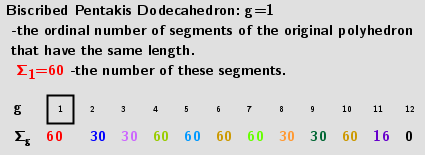# Rhombicosidodecahedron from Biscribed Pentakis Dodecahedron for the case of trisection of its 1st-order segments

A polyhedron is constructed whose V=120 vertices are the points of the trisection of the segments the same length 1st-order (g=1) of the Biscribed Pentakis Dodecahedron. Geometric Constructions are in Applet: Series of polyhedra obtained by trisection (truncation) different segments of the original polyhedron, and the resulting polyhedra in Applet: Serie of polyhedra obtained by trisection (truncation) segments of the Biscribed Pentakis Dodecahedron.## 2. Coloring edges and faces of polyhedra

If we assume that all quadrilaterals lie in the same plane, then our polyhedron approximately looks like Rhombicosidodecahedron : https://robertlovespi.net/2014/06/02/zonish-versions-of-the-rhombicosidodecahedron/﻿ This polyhedron based on adding zones along the five-fold symmetry axes of a rhombicosidodecahedron. All its edges are the same length, and its 62 faces include 20 triangles, 12 regular pentagons and 30 elongated octagons.